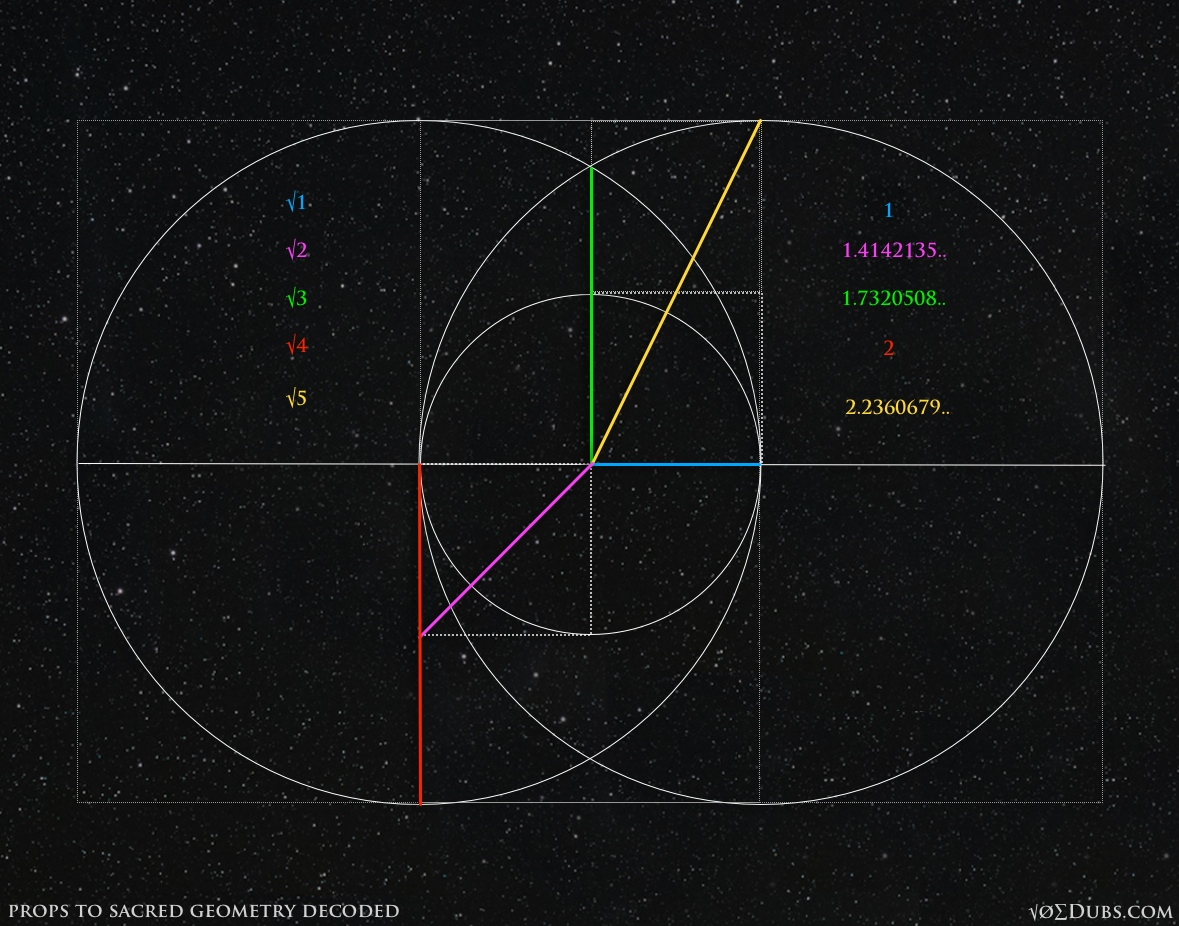## Square Roots & StuffSquare roots.  That sounds scary because there’s math involved.  Well don’t worry, this stuff’s easy.  Those scary numbers that look like √2, √3, and √5 are actually really simple concepts to understand. √2 or “the square root of two” is simply the cross section of a square when the sides equal one.  The square root of five is the cross section of a double square.  And the square root of three is the number formed by the vesica piscis. The square root of two is 1.414… because 1.414..  x  1.414.. = 2 1.732..  x  1.732.. = 3         So the square root of three is 1.732… 2.236..  x  2.236.. = 5       The square root of five is 2.236… The square root of one is one because 1 x 1 = 1   The square root of four is two because 2…

9